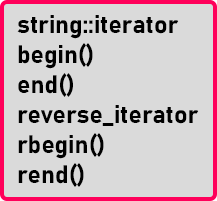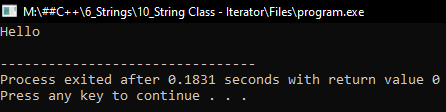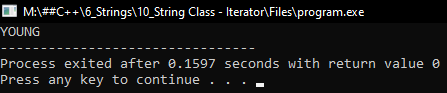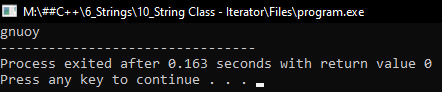# String Iterator in C++

## String Iterator in C++ with Examples:

In this article, I am going to discuss String Iterator in C++ Language with examples. Please read our previous article, where we discussed Substring, Compare, and Operators of String Class in C++ with examples.

##### String Iterator in C++:

Iterators are used for traversing or accessing all the characters of a string. Different iterators are available for string class.string::iterator allows us to access a string in the forward direction from left to right. reverse_iterator allows us to access a string in the backward direction that is from right to left. So, the iterator object will look like a pointer to a character in a string. By using this iterator, we can either read the alphabet or a character as well as we can mortify it.

##### How to access this

Let us see how to access this with examples.

string str = “Hello”;

Here we have declared the string object and now we should have an object of type iterator,

string::iterator it;

This is how we can declare the iterator. Now we can assign it by writing it as

it = str.begin();

This is the ‘begin()’ function and this gives the beginning index of this string. Similarly, we have ‘end()’ as

it = str.end();

This gives the ending index of a string. If we have to iterate all the characters in a string then we can use ‘for’ loop as

for(it = str.begin(); it != str.end(); it++){}

So, until ‘it’ reaches the end, ‘it’ will be going on moving to the next letter and it will be accessing all the letters. Suppose we want to print all the letters of a string then we will write,

```for(it = str.begin(); it != str.end(); it++){
cout << *it;
}```

Here we are writing ‘*it’, as we discuss that ‘it’ iterator will act as a pointer so we should dereference it for accessing a character. In this way, we can access all the characters one by one.

In the same way, we can use ‘reverse_iterator’ also. That will start from the end of a string i.e. ‘rbegin()’ and when it reaches the beginning of string i.e. ‘rend()’ will be true. ‘rbegin()’ and ‘rend()’ is reverse begin and reverse end. Now let us see all these functions in the C/C++ program.

##### Program 1:
```#include <iostream>
#include <string.h>
using namespace std;
int main()
{
string str = "Hello";
string::iterator it;

for (it = str.begin (); it != str.end (); it++)
{
cout << *it;
}

cout << endl;
return 0;
}
```
###### Output:##### Program 2:
```#include <iostream>
#include <string.h>
using namespace std;
int main()
{
string str = "young";
string::iterator it;

for (it = str.begin (); it != str.end (); it++)
{
*it = *it - 32;
}

cout << str;
}
```
###### Output:##### Program 3:
```#include <iostream>
#include <string.h>
using namespace std;
int main()
{
string str = "young";
string::reverse_iterator it;
for (it = str.rbegin (); it != str.rend (); it++)
{
cout << *it;
}
}
```
###### Output:##### Program 4:
```#include <iostream>
#include <string.h>
using namespace std;
int main()
{
string str = "young";
for (int i = 0; str[i] != '\0'; i++)
{
str[i] = str[i] - 32;
}
cout << str;
}
```
###### Output: